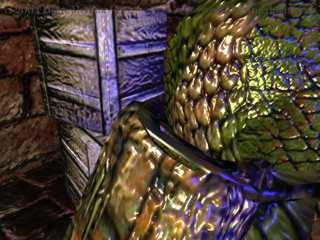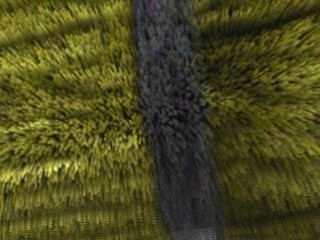Programmable shaders add a new dimension to graphics rendering by allowing the transform, lighting, and rendering functionality to be modified at runtime on a vertex and pixel basis. A shader is a small program that runs for every vertex, or every pixel that is rendered on the screen. It can control the vertex position, color and normal, the texture coordinates, as well as the pixel color and brightness, dependent on the influence of light, textures or arbitrary C-Script variables. This gives the user a new level of dynamic flexibility over the way that pixels are rendered. Vertex and pixel shaders can be used to create realistic water ripples, render cartoon style, cover models with fur, or control the lava flow of a volcano (screenshots by users on the Gamestudio Shader Forum).Normalmapping shader Fur shader

Shaders come in two flavors: vertex shaders manipulate mesh position vertices, pixel shaders manipulate texture pixels. The shader code is loaded into the graphics card memory and plugged directly into the graphics pipeline. Shader code is in assembly, however nowadays a higher level 'C' type language - HLSL, aka Cg - is used that is compiled down to the assembly and makes shaders much easier to program.

A shader description contains register declarations and shader instructions. Pixel and vertex shaders can be defined as part of the material effect script, by using the VertexShader and PixelShader keywords.

### Example for a vertex shader:

```float4x4 matWorldViewProj : WORLDVIEWPROJ;
float4 vecTime;

void vs_flicker_red(
in float4 iPos : POSITION,
in float2 iTex0 : TEXCOORD0,
in float2 iTex1 : TEXCOORD1,
out float4 oPos : POSITION,
out float4 oDiffuse: COLOR0,
out float2 oTex0 : TEXCOORD0,
out float2 oTex1 : TEXCOORD1)
{
oPos = mul(iPos,matWorldViewProj);
oTex0 = iTex0;
oTex1 = iTex1;
oDiffuse.r = fmod(vecTime.w * 0.1,1.0);
oDiffuse.g = 0.0;
oDiffuse.b = 0.0;
oDiffuse.a = 1.0;
}

technique flicker
{
pass p0
{
}
}```

Shader programming is non-trivial and requires some knowledge in vector and matrix algebra. However, once a shader effect is defined in a proper way, it can be used by everyone without knowledge of its internal works. A good introduction into writing shaders for Gamestudio is the Introduction to Shader Programming (see below). The shader language reference is contained in the DirectX 9 documentation. There are several books about shader programming. For an example how to write reusable shaders, look into the mtlFX shader library in the template_6\code folder.

Recommended tutorials and books:

Here is some basic information for shader programming:

### Vertex format

All geometry and model vertices use three UV coordinate sets for texture, lightmap/detail map, and shader tangents, in the following format:
```typedef struct {
float x,y,z;   // position   float nx,ny,nz; // normal
float tu1,tv1; // coordinate set 1, for texture or shadow map
float tu2,tv2; // coordinate set 2, for texture or detail map
float tx3,ty3,tz3,tw3; // coordinate set 3, for tangent or other purposes (A7.05 and above only)
} D3DVERTEX;

#define D3DFVF_D3DVERTEX (D3DFVF_XYZ|D3DFVF_NORMAL|D3DFVF_TEX3|D3DFVF_TEXCOORDSIZE2(0)|D3DFVF_TEXCOORDSIZE2(1)|D3DFVF_TEXCOORDSIZE4(2))```
Please note that the tw3 coordinate, which contains the orientation of the binormal, is only available in  A7.05  and above. A6 up to A7.4 only had 3 values at the third UV coordiate set. For correct handedness, multiply the binormal by tangent.w.

### The default.fx shader functions collection

The most common shader functions are contained in the template_6\code\default.fx file and can just be included in any shader that you use. This not only makes the shader code much shorter, it also avoids errors and allows adjustments that automatically affect all shaders. The shaders that come with Gamestudio all use the functions from the default.fx file, and you should make it a habit to use those functions for your shaders also. default.fx contains the following functions:
`float4 DoTransform(float4 Pos);  // vertex transformation to projection space - required for every shader`
`float4 DoPos(float4 Pos);		  // vertex transformation to world space`
`float4 DoView(float4 Pos);      // vertex transformation to view space`
`float4 DoNormal(float4 N);      // normal transformation to world space`
`float DoFog(float4 Pos);        // calculates the fog at position Pos`
`float4 DoSunLight(float3 N);    // calculates the sun light reflected by a surface with normal N`
```float4 DoPointLight(float3 P, float3 N, float4 vLightPos, float4 vLightColor);
// calculates the dynamic light from the given light source position and color
// reflected by a surface with normal N at position P. Used to sum up dynamic lights at a certain position.```
`void CreateTangents(float3 inNormal,float3 inTangent);// calculates matTangent, the tangent - binormal - normal matrix. Used for all further tangent space transformations.`
`float3 DoTangent(float3 Vector);	// transforms the vector to tangent space`

The usage of default.fx can be seen in the mtlFX shader library.

DX8 vertex shaders require a small modification for running under DirectX 9 and above. The following declaration in assembler shader language:
```decl
{
stream 0;
float v0; //position
float v3; //normal
float v7; //uv 1
float v8; //uv 2
}```

has to be replaced by a DX9 declaration:

```dcl_position v0
dcl_normal v3
dcl_texcoord0 v7
dcl_texcoord1 v8```

C   P